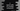# C program to print a square table of a number using pow()### C program to print a square table of a number using pow() :

In this tutorial, we will learn how to print one square table using pow() function. The user will enter one number and the program will print the square table for that number. Before explaining the program, let me explain to you how pow() works :

### pow() function in C :

The pow() function takes two parameters. We can define this function as double pow(double i,double j). Pass two numbers i and j to this function and it will calculate the value of i to the power j and returns the result. For example, pow(2,3) will return 8. The return value is a float. We are using the pow() function in the below program. Let’s take a look :

### C Program :

``````#include <stdio.h>
#include <math.h>

int main()
{
//1
int no, i;

//2
printf("Enter the number : ");
scanf("%d", &no);

//3
for (i = 1; i <= 10; i++)
{
printf("%d ^ %d = %.0f\n", no, i, pow(no, i));
}
}``````

### Explanation :

The commented numbers in the above program denote the step number below :

1. Create two integer variables no and i. no is used to hold the user input number and the variable i to use in the loop.
2. Ask the user to enter the number. Read it and save it in no variable.
3. Run one for loop starting from 1 to 10 . For each time, print the power of the no using pow(no,i) function.

### Sample Output :

``````Enter the number : 5
5 ^ 1 = 5
5 ^ 2 = 25
5 ^ 3 = 125
5 ^ 4 = 625
5 ^ 5 = 3125
5 ^ 6 = 15625
5 ^ 7 = 78125
5 ^ 8 = 390625
5 ^ 9 = 1953125
5 ^ 10 = 9765625

Enter the number : 2
2 ^ 1 = 2
2 ^ 2 = 4
2 ^ 3 = 8
2 ^ 4 = 16
2 ^ 5 = 32
2 ^ 6 = 64
2 ^ 7 = 128
2 ^ 8 = 256
2 ^ 9 = 512
2 ^ 10 = 1024

Enter the number : 1
1 ^ 1 = 1
1 ^ 2 = 1
1 ^ 3 = 1
1 ^ 4 = 1
1 ^ 5 = 1
1 ^ 6 = 1
1 ^ 7 = 1
1 ^ 8 = 1
1 ^ 9 = 1
1 ^ 10 = 1``````